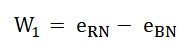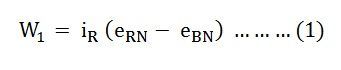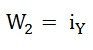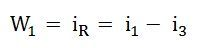# Two Wattmeter Method of Power Measurement

Two Wattmeter Method can be employed to measure the power in a 3 phase, three-wire star or delta connected the balanced or unbalanced load.

In two wattmeter method, the current coils of the wattmeter are connected with any two lines, say R and Y and the potential coil of each wattmeter is joined on the same line, the third line i.e. B as shown below in figure (A):The total instantaneous power absorbed by the three loads Z1, Z2 and Z3, is equal to the sum of the powers measured by the two wattmeters, W1 and W2.

Contents:

## Measurement of Power by Two Wattmeter Method in Star Connection

Considering the above figure (A) in which Two Wattmeter W1 and W2 are connected, the instantaneous current through the current coil of Wattmeter, W1 is given by the equation shown below:The instantaneous potential difference across the potential coil of Wattmeter, W1 is given as:Instantaneous power measured by the Wattmeter, W1 isThe instantaneous current through the current coil of Wattmeter, W2 is given by the equation:The instantaneous potential difference across the potential coil of Wattmeter, W2 is given as:Instantaneous power measured by the Wattmeter, W2 is:Therefore, the total power measured by the two wattmeters W1 and W2 will be obtained by adding the equation (1) and (2).Where, P – the total power absorbed in the three loads at any instant.

## Measurement of Power by Two Wattmeter Method in Delta Connection

Considering the delta connected circuit shown in the figure below:The instantaneous current through the coil of the wattmeter, W1 is given by the equation:Instantaneous power measured by the Wattmeter, W1 will be:Therefore, the instantaneous power measured by the wattmeter, W1 will be given as:The instantaneous current through the current coil of the Wattmeter, W2 is given as:The instantaneous potential difference across the potential coil of wattmeter, W2 :Therefore, the instantaneous power measured by Wattmeter, W2 will be:Hence, to obtain the total power measured by the two wattmeter the two equations, i.e. equation (3) and (4) has to be added.Where P is the total power absorbed in the three loads at any instant.

The power measured by the Two Wattmeter at any instant is the instantaneous power absorbed by the three loads connected in three phases. In fact, this power is the average power drawn by the load since the Wattmeter reads the average power because of the inertia of their moving system.

### 19 thoughts on “Two Wattmeter Method of Power Measurement”

1. The motor can run in reverse mode by reversing the line connections of either the main winding or the starting winding.

1. 1. Two wattmeter method measures the three phase power.
2. Useful for both the balanced and unbalanced loads.

2. will there be any difference in result if we use 2wattmeter method vs 3 wattmeter method on same 11kV circuit?with same load?

3. I think it is “Instantaneous voltage measured by the Wattmeter, W1 will be

W sub 1 = e sub RB”

4. explain relation between single phase power and three phase power (show the equations)

5. Is there any serious errors in measurement of power through watt metre of 1 and 2 it there any how to minimise it

6. While measuring power in a 3 phase load by 2 wattmeter methodbthe reading of 2 wattmeter are equal nd opposite why

7. Does the two wattmeter method of power measurement applicable for a 3 phase, 4 phase unbalanced load.if not how to measure the total power absorbed by three unequal load